# Finding Hexadecimal Value for the Decimal number from above table: In the table given above, the number of rows represent the first hexadecimal digit (left Hexadecimal digit) and the number of columns represent the second hexadecimal digit (right hexadecimal digit) of the hexadecimal number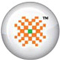PRO DATA DOCTOR it | es | pt | fr | de | jp | kr | cn | ru | nl | gr

Thus we get hexadecimal number 03ABE H, equivalent to the decimal number 15038 and in this way we are back with the original number. That is what we should expect.

The table given next can help to get the quick search of Hexadecimal number to decimal number conversion and vice-versa from the range of 0 to 255 decimal numbers.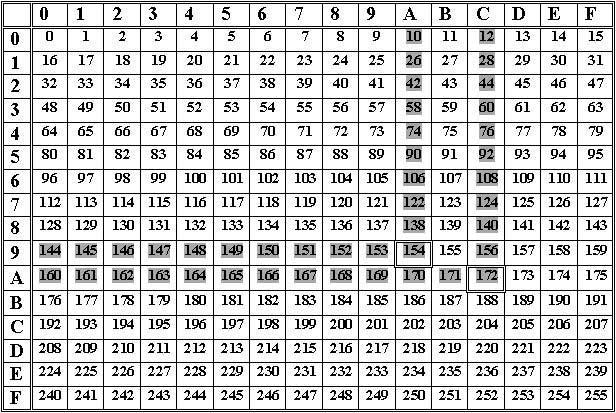In this Square table there are 16 rows, starting from 0 to A and there are 16 columns also starting from 0 to A. From this table you can find the decimal value of any hexadecimal number that is in between the range of 0H to FFH. It means that the decimal value of the number should be in between the range of 0 to 255 decimal numbers.

• Finding Decimal value for Hexadecimal number from above table: In the table given above, the number of rows represent the first hexadecimal digit (left Hexadecimal digit) and the number of columns represent the second hexadecimal digit (right hexadecimal digit) of the hexadecimal number.

Let we have any hexadecimal number say ACH, to be converted into the equivalent decimal number. Then we shall see the decimal value in the Cth column of the Ath row in the table and get the decimal value 172, which is the equivalent decimal number for the hexadecimal number ACH.

• Finding Hexadecimal Value for the Decimal number from above table: In the table given above, the number of rows represent the first hexadecimal digit (left Hexadecimal digit) and the number of columns represent the second hexadecimal digit (right hexadecimal digit) of the hexadecimal number thus if you have any decimal number to be converted into equivalent hexadecimal number, search the number in the table and find the equivalent hexadecimal value as follows:

Hex Value for the Decimal Number =
(Row Number)(Column Number)

For example if you want to find the equivalent hexadecimal value number for the decimal number 154, see the location of the number in the table. The number 154 is in the 9th row and Ath column of the table. Thus the equivalent hexadecimal value for the decimal number 154 is 9AH.

Sample Chapters from book DATA RECOVERY WITH AND WITHOUT PROGRAMMING by Author Tarun Tyagi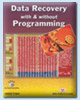Publishers of the Book Number of Pages ISBN Price of the Book BPB Publications, New Delhi, India 540 81-7656-922-4 \$69.00 (Including Shipping Charges, Cost of Book and Other expenses, Free Source Code CD included with the Book)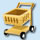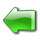page 1 | 2 | 3 | 4 | 5 | 6 | 7 | 8 | 9 | 10 | 11 | 12 | 13 | 14 | 15 | 16 | 17 | 18 | 19 | 20

 page 21 | 22 | 23 | 24 | 25 | 26 | 27 | 28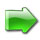© Copyright 2002-2005 DataDoctor.Biz
 Website Data Recovery | recuperación de Datos | Récupération de données | Datenrettung | Recupero dati | データ復旧 | 데이터 복구 | 数据恢复 | Восстановление данных | De terugwinning van gegevens | Ανάκτηση δεδομένων Sitemap Site map1 2 3 4 | Spanish1 2 3 | French1 2 3 | German1 2 3 | Italian1 2 3 | Portuguese1 2 3 | Japanese1 2 3 | Korean1 2 3 | Chinese1 2 3 | Russian1 2 3 | Dutch1 2 3 | Greek1 2 3 Data Recovery Book English | Spanish | French | German | Italian | Portuguese | Japanese | Korean | Chinese | Russian | Dutch | Greek